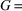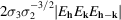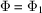International Tables for Crystallography (2006). Vol. B. ch. 2.2, pp. 210-234   | 1 | 2 | https://doi.org/10.1107/97809553602060000555

## Contents

• 2.2. Direct methods  (pp. 210-234)
• 2.2.1. List of symbols and abbreviations  (p. 210) | html | pdf |
• 2.2.2. Introduction  (p. 210) | html | pdf |
• 2.2.3. Origin specification  (pp. 210-215) | html | pdf |
• 2.2.4. Normalized structure factors  (pp. 215-217) | html | pdf |
• 2.2.4.1. Definition of normalized structure factor  (pp. 215-216) | html | pdf |
• 2.2.4.2. Definition of quasi-normalized structure factor  (p. 216) | html | pdf |
• 2.2.4.3. The calculation of normalized structure factors  (pp. 216-217) | html | pdf |
• 2.2.4.4. Probability distributions of normalized structure factors  (p. 217) | html | pdf |
• 2.2.5. Phase-determining formulae  (pp. 217-225) | html | pdf |
• 2.2.5.1. Inequalities among structure factors  (pp. 217-218) | html | pdf |
• 2.2.5.2. Probabilistic phase relationships for structure invariants  (p. 218) | html | pdf |
• 2.2.5.3. Triplet relationships  (pp. 218-219) | html | pdf |
• 2.2.5.4. Triplet relationships using structural information  (p. 220) | html | pdf |
• 2.2.5.5. Quartet phase relationships  (pp. 220-222) | html | pdf |
• 2.2.5.6. Quintet phase relationships  (pp. 222-223) | html | pdf |
• 2.2.5.7. Determinantal formulae  (pp. 223-224) | html | pdf |
• 2.2.5.8. Algebraic relationships for structure seminvariants  (p. 224) | html | pdf |
• 2.2.5.9. Formulae estimating one-phase structure seminvariants of the first rank  (pp. 224-225) | html | pdf |
• 2.2.5.10. Formulae estimating two-phase structure seminvariants of the first rank  (p. 225) | html | pdf |
• 2.2.6. Direct methods in real and reciprocal space: Sayre's equation  (pp. 225-227) | html | pdf |
• 2.2.7. Scheme of procedure for phase determination  (pp. 227-228) | html | pdf |
• 2.2.8. Other multisolution methods applied to small molecules  (pp. 228-230) | html | pdf |
• 2.2.9. Some references to direct-methods packages  (p. 230) | html | pdf |
• 2.2.10. Direct methods in macromolecular crystallography  (pp. 231-234) | html | pdf |
• 2.2.10.1. Introduction  (p. 231) | html | pdf |
• 2.2.10.2. Ab initio direct phasing of proteins  (pp. 231-232) | html | pdf |
• 2.2.10.3. Integration of direct methods with isomorphous replacement techniques  (p. 232) | html | pdf |
• 2.2.10.4. Integration of anomalous-dispersion techniques with direct methods  (pp. 232-234) | html | pdf |
• 2.2.10.4.1. One-wavelength techniques  (p. 233) | html | pdf |
• 2.2.10.4.2. The SIRAS, MIRAS and MAD cases  (pp. 233-234) | html | pdf |
• References | html | pdf |
• Figures
• Fig. 2.2.4.1. Probability density functions for cs. and ncs. crystals  (p. 216) | html | pdf |
• Fig. 2.2.4.2. Cumulative distribution functions for cs. and ncs. crystals  (p. 216) | html | pdf |
• Fig. 2.2.5.1. Curves of (2.2.5.6) for some values of(p. 219) | html | pdf |
• Fig. 2.2.5.2. Variance (in square radians) as a function of α  (p. 219) | html | pdf |
• Fig. 2.2.5.3. Distributions (2.2.5.18) (solid curve) and (2.2.5.20) (dashed curve) for the indicatedvalues in three typical cases  (p. 221) | html | pdf |
• Fig. 2.2.7.1. The form of w as given by (2.2.7.2)  (p. 227) | html | pdf |
• Tables
• Table 2.2.3.1. Allowed origin translations, seminvariant moduli and phases for centrosymmetric primitive space groups  (p. 211) | html | pdf |
• Table 2.2.3.2. Allowed origin translations, seminvariant moduli and phases for noncentrosymmetric primitive space groups  (pp. 212-213) | html | pdf |
• Table 2.2.3.3. Allowed origin translations, seminvariant moduli and phases for centrosymmetric non-primitive space groups  (p. 214) | html | pdf |
• Table 2.2.3.4. Allowed origin translations, seminvariant moduli and phases for noncentrosymmetric non-primitive space groups  (pp. 214-215) | html | pdf |
• Table 2.2.4.1. Moments of the distributions (2.2.4.4) and (2.2.4.5)  (p. 217) | html | pdf |
• Table 2.2.5.1. List of quartets symmetry equivalent toin the class mmm   (p. 222) | html | pdf |
• Table 2.2.8.1. Magic-integer sequences for small numbers of phases ( n ) together with the number of sets produced and the root-mean-square error in the phases  (p. 229) | html | pdf |